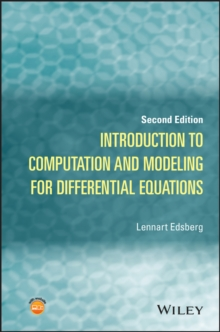Supporting your high street Find out how »
• My Account# Introduction to Computation and Modeling for Differential Equations Hardback

#### Description

Uses mathematical, numerical, and programming tools to solve differential equations for physical phenomena and engineering problems Introduction to Computation and Modeling for Differential Equations, Second Edition features the essential principles and applications of problem solving across disciplines such as engineering, physics, and chemistry.

The Second Edition integrates the science of solving differential equations with mathematical, numerical, and programming tools, specifically with methods involving ordinary differential equations; numerical methods for initial value problems (IVPs); numerical methods for boundary value problems (BVPs); partial differential equations (PDEs); numerical methods for parabolic, elliptic, and hyperbolic PDEs; mathematical modeling with differential equations; numerical solutions; and finite difference and finite element methods. The author features a unique Five-M approach: Modeling, Mathematics, Methods, MATLAB(R), and Multiphysics, which facilitates a thorough understanding of how models are created and preprocessed mathematically with scaling, classification, and approximation and also demonstrates how a problem is solved numerically using the appropriate mathematical methods. With numerous real-world examples to aid in the visualization of the solutions, Introduction to Computation and Modeling for Differential Equations, Second Edition includes: * New sections on topics including variational formulation, the finite element method, examples of discretization, ansatz methods such as Galerkin s method for BVPs, parabolic and elliptic PDEs, and finite volume methods * Numerous practical examples with applications in mechanics, fluid dynamics, solid mechanics, chemical engineering, heat conduction, electromagnetic field theory, and control theory, some of which are solved with computer programs MATLAB and COMSOL Multiphysics(R) * Additional exercises that introduce new methods, projects, and problems to further illustrate possible applications * A related website with select solutions to the exercises, as well as the MATLAB data sets for ordinary differential equations (ODEs) and PDEs Introduction to Computation and Modeling for Differential Equations, Second Edition is a useful textbook for upper-undergraduate and graduate-level courses in scientific computing, differential equations, ordinary differential equations, partial differential equations, and numerical methods. The book is also an excellent self-study guide for mathematics, science, computer science, physics, and engineering students, as well as an excellent reference for practitioners and consultants who use differential equations and numerical methods in everyday situations.

#### Information

£78.95

£66.85